Integral Calculus -7

# Integral Calculus -7

Test Description

## 20 Questions MCQ Test | Integral Calculus -7

Integral Calculus -7 for Mathematics 2023 is part of Mathematics preparation. The Integral Calculus -7 questions and answers have been prepared according to the Mathematics exam syllabus.The Integral Calculus -7 MCQs are made for Mathematics 2023 Exam. Find important definitions, questions, notes, meanings, examples, exercises, MCQs and online tests for Integral Calculus -7 below.
Solutions of Integral Calculus -7 questions in English are available as part of our course for Mathematics & Integral Calculus -7 solutions in Hindi for Mathematics course. Download more important topics, notes, lectures and mock test series for Mathematics Exam by signing up for free. Attempt Integral Calculus -7 | 20 questions in 60 minutes | Mock test for Mathematics preparation | Free important questions MCQ to study for Mathematics Exam | Download free PDF with solutions
 1 Crore+ students have signed up on EduRev. Have you?
Integral Calculus -7 - Question 1

### What is the limit of the product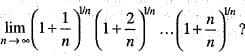Detailed Solution for Integral Calculus -7 - Question 1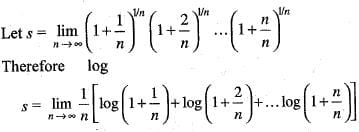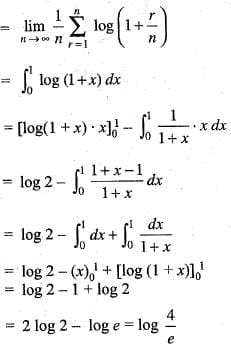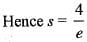Integral Calculus -7 - Question 2

### The length of the cycloid with parametric equation x(t) = (t + sin t), y(t) = (1 - cos t) between (0, 0) and (π, 2) is:

Detailed Solution for Integral Calculus -7 - Question 2

The given curve is
x(t) = (t + sin t), y(t) = (1 - cos t)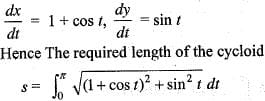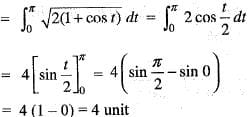Integral Calculus -7 - Question 3

### Which of the following is/are true? 1.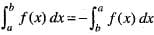2.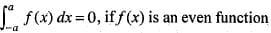3.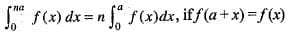4.  If f(x) ≥ φ(x) and both functions are integrable in [a, b]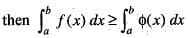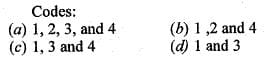Integral Calculus -7 - Question 4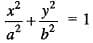is revolved about major and minor axis respectively. Then the ratio of these solids made by two revolutions is:

Detailed Solution for Integral Calculus -7 - Question 4

Case (i): When ellipse is rotated about major axis:

Take a small disc at a length of x from the centre of thickness dx. Then the volume of solid obtained by rotation will be ∫(−a to a)​(Area)dx

Area of disc =πr2

r can be calculated from the equation of ellipse as

x2/a2​ + r2/b= 1

⇒ r= b2(1−x2/a2​) = b2/a2​(a2−x2)

∴Volume of major axis​ = ∫(−a to a)​πa2/b2​(a2−x2)dx

= [πb2x − πb2x3/3a2​](−a to a)

​= 4/3​πab2

Case (ii): When ellipse is rotated about minor axis:

Following similar procedure as case (i),

r= a2/b2​(b− y2)

In this case, the area will be integrated w.r.t dy as it is rotated about the Y-axis.

∴Volume of minor axis​ = ∫(−a to a)​πa2/b2​(b2−y2)dx

= [πa2y − πa2y3/3b2​](−a to a)

​= 4/3​πab2

= b/a

Integral Calculus -7 - Question 5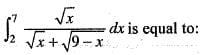Detailed Solution for Integral Calculus -7 - Question 5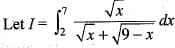...(i)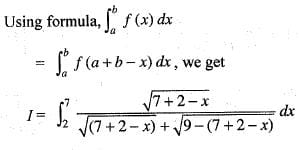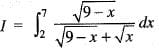...(ii)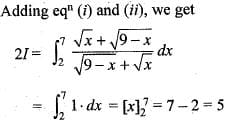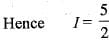Integral Calculus -7 - Question 6

The surface area of the segment of a sphere of radius a and height h is given by:

Detailed Solution for Integral Calculus -7 - Question 6

Let the sphere be generated by the revolution about the x - axis of the circle
x2 + y2=a2 ...(i)
Let OA =a, OC = b and OB = b + h
Hence The required surface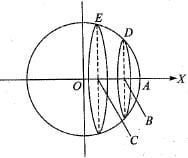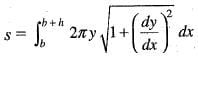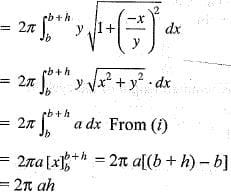Integral Calculus -7 - Question 7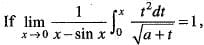then the value of a is:

Detailed Solution for Integral Calculus -7 - Question 7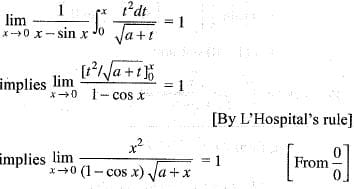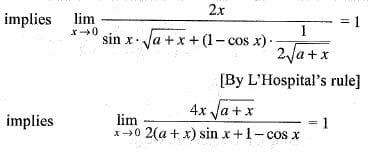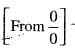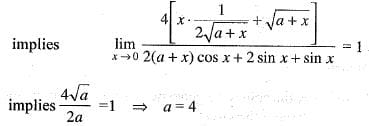Integral Calculus -7 - Question 8

The moment of inertia about the axis of y of the region in the xy-plane bounded by y = 4 - x2 and the x-axis, is, proportional to

Detailed Solution for Integral Calculus -7 - Question 8

The given curve is y = 4x2
Hence Required moment of inertia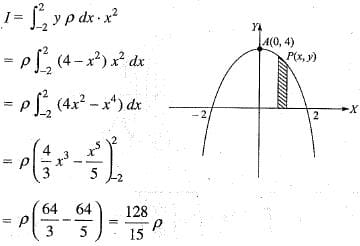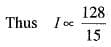Integral Calculus -7 - Question 9

The value of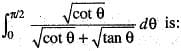Detailed Solution for Integral Calculus -7 - Question 9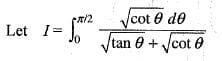...(i)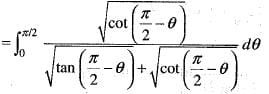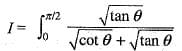...(ii)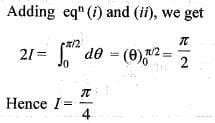Integral Calculus -7 - Question 10

Match the list-I with list-II: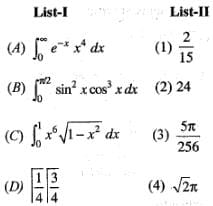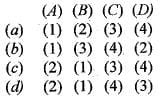Detailed Solution for Integral Calculus -7 - Question 10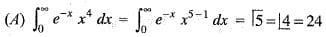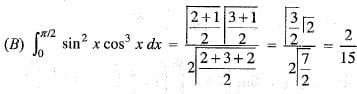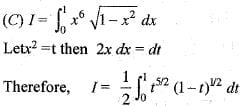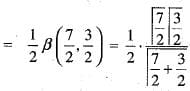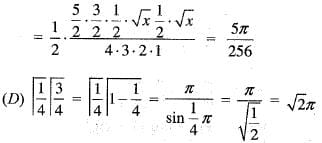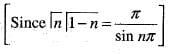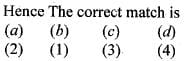Integral Calculus -7 - Question 11

The length of the arc of the curve y = loge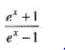Detailed Solution for Integral Calculus -7 - Question 11

Given that
y = loge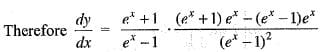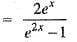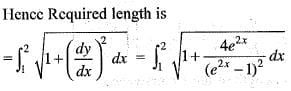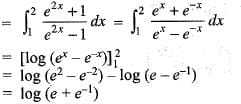Integral Calculus -7 - Question 12

The intrinsic equations of the cardioids r = a (1 - cos θ) and r = a(1 + cos θ) measured from the pole are:

Detailed Solution for Integral Calculus -7 - Question 12

The equations of given cardioids are
r= a (l -cos θ)    ...(i)
r= a (1 + cos θ)   ...(ii)
Intrinsic equation for cardioid (i):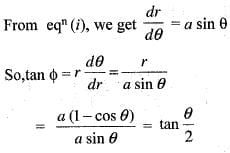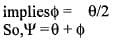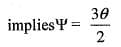...(iii)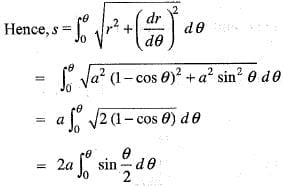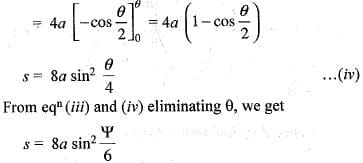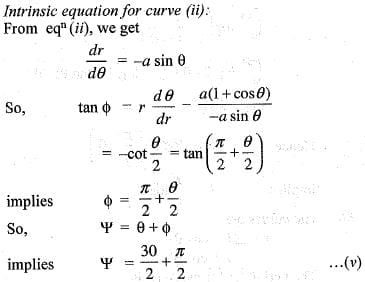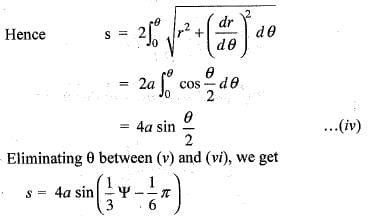Integral Calculus -7 - Question 13

Consider the Assertion (A) and Reason (R) given below:

Assertion (A)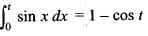Reason (R) - sin x is continuous in any closed interval [ 0 ,t] .

Integral Calculus -7 - Question 14

The value of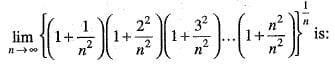Detailed Solution for Integral Calculus -7 - Question 14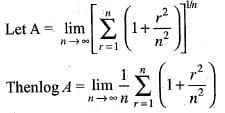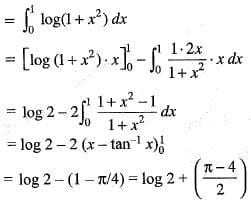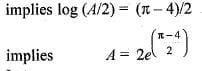Integral Calculus -7 - Question 15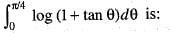Detailed Solution for Integral Calculus -7 - Question 15

Let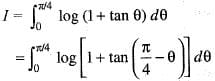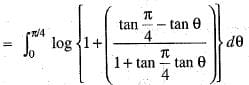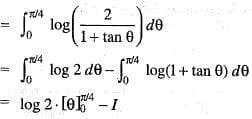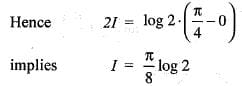Integral Calculus -7 - Question 16

The perimeters of the cardioids r = a (1 - cos θ) and r = a (1 + cos θ) differ by:

Detailed Solution for Integral Calculus -7 - Question 16

The curves are
r = a(1 - cos θ)     ...(i)
r = a(1 + cos θ)    ...(ii)
The perimeter for curve (i) is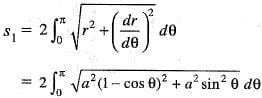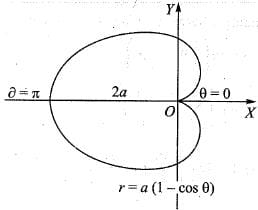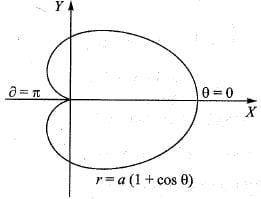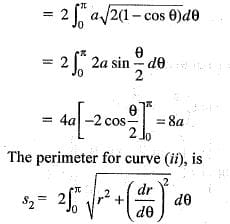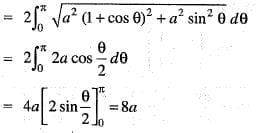Hence s1 - s2 = 8a - 8a = 0.

Integral Calculus -7 - Question 17

The length of the arc of the curve 6xy = x4 + 3 from x = 1 to x = 2 is:

Detailed Solution for Integral Calculus -7 - Question 17

The given curve is
6xy = x4 + 3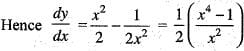Thus Length of the arc between x
= 1 and x = 2 is: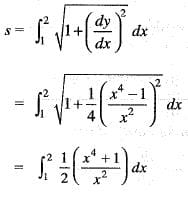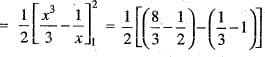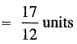Integral Calculus -7 - Question 18

The area of the region in the first quadrant bounded by the y-axis and curves y = sin x and y = cos x is:

Detailed Solution for Integral Calculus -7 - Question 18

The given curves are
y = sin x   ...(i)
y = cos x   ...(ii)
The curves intersect at a point B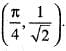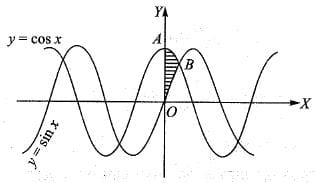Hence Required area = Area OABO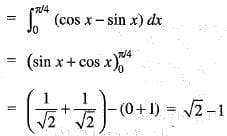Integral Calculus -7 - Question 19

The line y = x + 1 is revolved about x-axis. The volume of solid of revolution formed by revolving the area covered by the given curve, x-axis and lines x = 0, x = 2 is:

Detailed Solution for Integral Calculus -7 - Question 19

The given curve is y = x + 1. This represents a straight line
Hence The required volume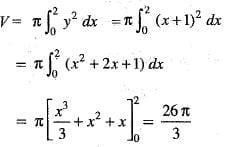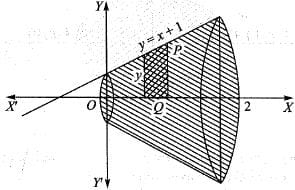Integral Calculus -7 - Question 20

The length of the complete cycloid x = a (θ + sin θ), y = a(1 - cos θ) is given by:

Detailed Solution for Integral Calculus -7 - Question 20

The equations of the curve are
x = a(θ + sin θ), y = a(1 - cos θ)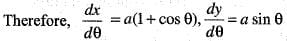Hence The entire length of the curve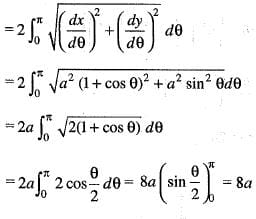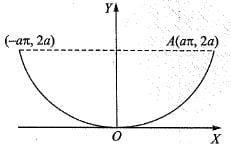Information about Integral Calculus -7 Page
In this test you can find the Exam questions for Integral Calculus -7 solved & explained in the simplest way possible. Besides giving Questions and answers for Integral Calculus -7, EduRev gives you an ample number of Online tests for practice(Scan QR code)